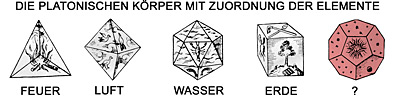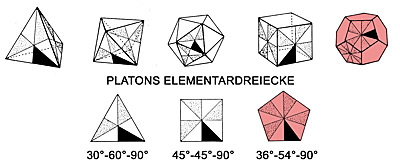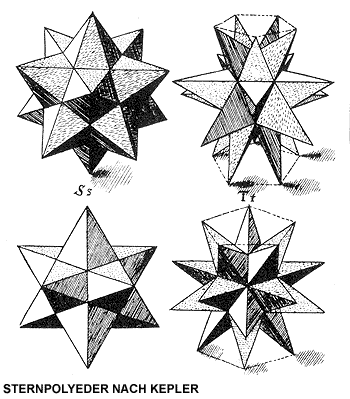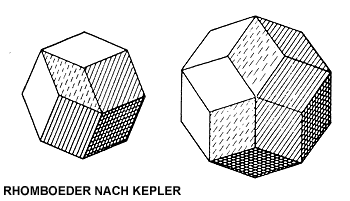The history of western science begins with Pythagoras and the Pythagoreans, a secret school which only transmitted its findings orally. This geometrical body of thought was essentially laid down by Plato in his dialogue "Timaeus". With Pythagoras "everything is number" - he tried to describe natural phenomena mathematically, to find numerical ratios in them. Here, we are first of all reminded of Pythagorean music theory, in which harmonious intervals are represented as proportions of whole numbers: the octave 2:1, the fifth 3:2, the fourth 4:3. This corresponds to the lengths of the vibrating strings of the monochord. These numerical ratios became axiomatic."With Plato, a field of knowledge can only be named science when it is possible to develop and classify the totality of its objects in a system. Just this succeeded in the case of sounds: the idea of a sound which differentiates by its relation to a higher, lower or equal sound receives an order through the axiomatically possible consonances. Then the consonant intervals fix all possible sounds of a scale systematically. Plato demanded corresponding forms of science for all areas, as geometry, stereometry and astronomy." (Böhme)

The epochal event was the discovery of the number in natural phenomena. Stereometry is in fact the basis of architecture.

Euclid defines the regular body as a figure which is limited by equal,equilateral and equiangular surfaces, That proves that there can be only five such solids, the so-called Platonic solids: tetrahedron, octahedron, hexahedron, dodecahedron and icosahedron, a finite list. Their vertices lie on the circumscribed sphere. To these "platonic" solids, Plato assigns the four elements: earth to the cube, water to the icosahedron, air to the octahedron, fire to the tetrahedron. The dodecahedron represents the world as a whole, the Cosmos.
He disassembles tetrahedron, octahedron, cube and icosahedron into elements, namely only two triangles, the sixths of an equilateral triangle and the eighth of a square, and in this way finds the elements of the physical world of his imagination.

For Plato it was a problem that five platonic solids were known at the time, although there were only four elements to correspond with them; so he assigned the dodecahedron with its non surface-filling pentagon as a face to the principle of entirety, space or cosmos, i.e. to the "ether" which, to me, appears to be a makeshift solution.

Furthermore, he reduced the faces of the octahedron, tetrahedron and the icosahedron via triadic symmetry, and that of the cube via tetradic symmetry into its only two so-called elementary triangles, again leaving the pentagon out of the system. This he describes in detail in his dialogue "Timaeus":

"Every plane surface consists of triangles. And all triangles are based on two, of which each has a right angle and two acute angles: in one triangle, two of the sides are equal and the two acute angles are halves of one right angle, in the other triangle, the two acute angles are inequal, as well as the two sides. These two types of triangle are the origin of fire, and form all other solids. (...)

In the following, we must now demonstrate from what properties the four most beautiful solids originate, dissimilar each to the other, though endowed with the property that one can be created from the other, should a number of them have disappeared. (...)

We must therefore direct our efforts to follow the neat symmetrical conjoining of these four types of solid, remarkable for their beauty, and thus to show that we have understood their nature."

Plato was not able to meet his own demand for a finite list; in fact, a solution for integrating a symmetry based on the number five was not realized until 1980, through the work of Penrose and Shechtman.

Archimedes discovered and listed solids with less strict symmetric demands, the so-called semiregular Archimedean solids, which were generated via equilateral, but different, surfaces. This again provided a finite list of thirteen - and only thirteen -polyhedrons.

More than 2000 years later, Kepler described two regular concave solids, the so-called star polyhedrons, of 12 or 30 vertices, and both rhombohedrons, which are generated by equal, equilateral, but not equiangular surfaces. The latter one formed the basis for quasicrystals, which were not discovered until 1984 by Shechtman.In his "Mysterium cosmographicum", Kepler combined the Platonic solids with each other and with their inscribed or circumscribed spheres to explain the distances of the orbits of planets known at that time.

"Nevertheless, the mathematical theory of perfect solids not only led to the first creative argument between philosophy, mathematics and art, but can also be considered a classic example of other finite theories. The aim to enumerate classes of structures and to classify them is generally one of the predominant subjects in science. Here, from the Pythagoreans, the way leads via Archimedes and Kepler directly to some of the most important results and the most fascinating problems in modern mathematics. " (Dress)
HISTORY